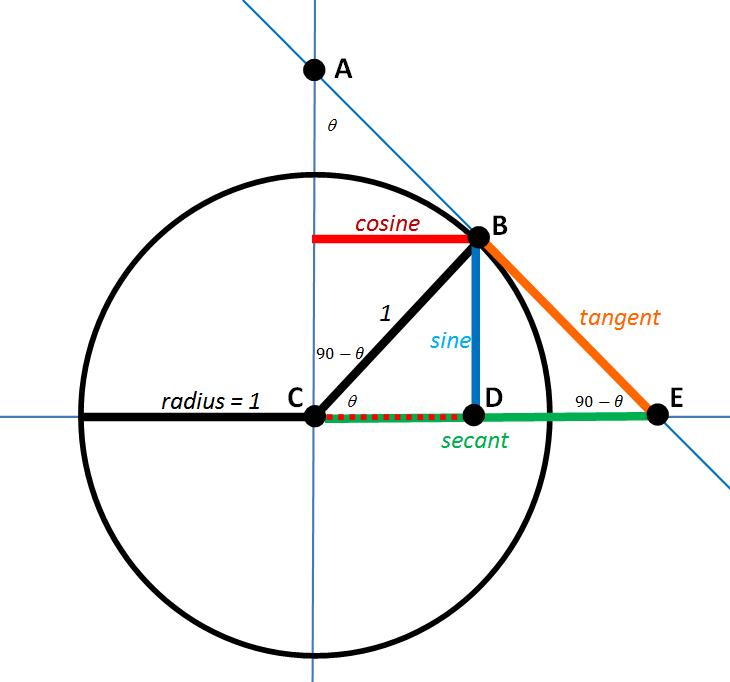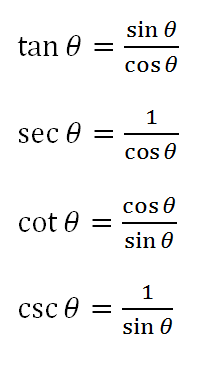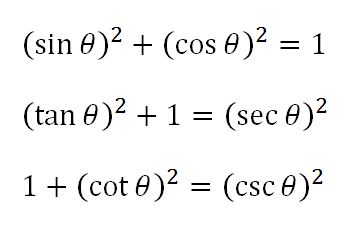# Trig Pictures, Part III

We almost have the whole picture in view.

Here’s where we left off:In this diagram, the secant line segment is adjacent to the central angle θ.  And the tangent line segment is opposite that same angle.

But what if we want to draw our secants and tangents relative to the central angle 90-θ, the complement?  Let’s add those segments to the diagram and call them the cosecant and the cotangent.EXERCISE #1

Find similar triangles and then use ratios to establish each of the following:(If you need help getting started, I did the first two of these for you in the last post.)

EXERCISE #2

Find right triangles and then apply the Pythagorean theorem to establish each of the following:Those two exercises reveal a lot of information all derived from that one diagram.  Once again…it’s better with pictures.

Comments are closed.